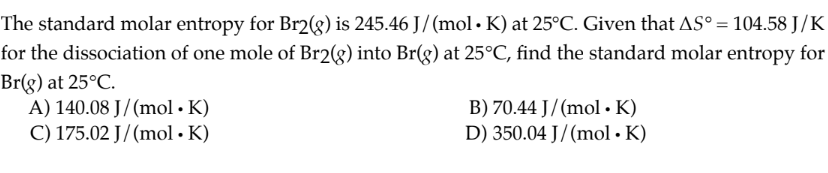# The standard molar entropy for Br2(g) is 245.46 J/(mol∙ K) at 25°C. Given that ∆S° = 104.58 J/K for the dissociation of one mole of Br2(g) into Br(g) at 25°C, find the standard molar entropy for Br(g) at 25°C. A) 140.08 J/(mol ∙ K) B) 70.44 J/(mol ∙ K) C) 175.02 J/(mol ∙ K) D) 350.04 J/(mol ∙ K)